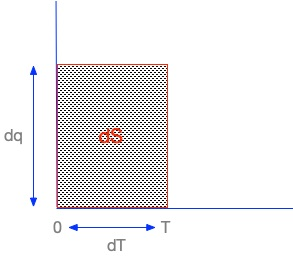# Chemistry

#### Chemistry 18: 4-5WebLecture

# Measuring Entropy

## Standard Entropy

In order to compare entropy values, we have to have some way of measuring entropy and a baseline to use for change. From the third law of thermodynamics, we know that at 0 K, a substance in a perfect crystalline state has zero entropy. For any temperature above 0 K, the entropy will depend on the amount of heat required to raise the substance to that temperature. As with standard heat capacities, we measure entropy amounts as energy at a given temperature for a given amount of the substance, in this case, moles. Since entropy depends on Kelvin temperatures, which are always postive, and positive amounts of added heat, entropy is always positive.

There are some general entropy behaviors we can note for different molecules as we add heat and entropy increases. Phase changes from solid to liquid and liquid to gas involve large quantities of heat, and entropy increases drastically with these state changes. Another general conclusion we can make is that larger molecules, or more complex molecules, have more microstates than smaller, simpler molecules, so they tend ot have larger entropies.

Entropy is measured as the heat added through a reversible process, one in which a system remains in thermodynamic equilibrium with its surroundings, responding nearly instantaneously to incremental changes in the environment. No real process is completely reversible, but many chemical reactions near equilibrium are close enough to reversibility that we can use this situation to measure entropy changes at standard temperature (25°C) and pressure (1atm). Standard entropy then is the heat required to raise the temperature of a substance from absolute zero to 25°C = 298 K. In formal terms, we have

which really means this:• We will raise the temperature of a substance from 0K to T in some number of steps equal to k.
• Each step raises the temperature a small amount dT.
• The entropy change dS for the kth step at temperature T equals the amount of heat energy dqk added to raise the temperature by dT.

So the area dq * dT = dS in the box is the change in entropy for the k = 1 step. If we add up the heat change amounts and temperature change amounts over the range from 0K to 298K, we get the total entropy change S required for that particular substance.

The S° amount has been determined for many substances. As with enthalpy H°, we can determine a change in entropy by subtracting the entropy of the products from the entropy of the reactants.

Using data in tables for S° amounts already determined, we can predict the entropy change for many reactions. Even where we do not know S°, we can predict the entropy change from enthalpy changes: ]

If we assume that a process is reversible and occurs at constant temperature and pressure, we can calculate the change in entropy of the environment of a reaction as a function of the change in enthalpy of the reaction system:

## Spontaneity, Entropy, and Enthalpy

We can now put entropy and enthalpy together and determine whether a reaction is likely to be spontaneous.

Enthalpy increases and is postive when a reaction is endothermic, and such reactions are likely to be non-spontaneous. Enthalpy decreases and is negative when a reaction is exothermic, and these exothermic reactions are likely to be spontaneous.

When entropy increases and is postive, a reaction is likely to be spontaneous. If it decreases and is negative, the reaction is likely to be non-spontaneous.

So now we put the two factors together. We have four possible situations:

Type Change in Enthalpy Change in Entropy Spontaneity
1 Decreasing ΔH < 0 Positive ΔS > 0 Always spontaneous at any temperature
2 Decreasing ΔH < 0 Negative ΔS < 0 Depends on values; spontaneous at lower temperatures
3 Increasing ΔH > 0 Positive ΔS > 0 Depends on values; spontaneous at higher temperatures
4 Increasing ΔH > 0 Negative ΔS < 0 Not spontaneous at any temperature

### Practice with the Concepts

Consider an endothermic reaction in which entropy is increasing. Is the reaction every spontaneous? If so, under what conditions?

### Discussion Questions

• Why is spontaneity of a reaction a concern for chemists?